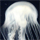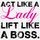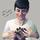General QuestionStatistics question: a binomial model can be approximated with what other model?

Asked by 717richboy (234) November 14th, 2012
7 responses
“Great Question” (0)

I’m not the best when it comes to stats, and I am confused about which model closely resembles the binomial model. Thanks in advance.Follow this questionSend to a friend!Topics: , ,
Observing members: 0Composing members: 0Normal distribution?

livelaughlove21 (15717)“Great Answer” (0)Well, we’re really not supposed to give away homework answers on Fluther, but I do know this one. I’m trying to think how to lead you to it without just telling you.

When you have very large samples sizes, there is a type of distribution that begins to behave similarly to a binomial distribution. There are some formulas: for large n, μ ≈ np and σ ≈ √(np(1-p)).

Got any ideas now?

Mariah (25876)“Great Answer” (1)I want to say normal distribution, but I’m still not 100% sure.

717richboy (234)“Great Answer” (0)Normal model*

717richboy (234)“Great Answer” (0)Right, so how can you be more confident in that answer? Look at my formulas. These are the formulas for approximating a binomial distribution: μ ≈ np and σ ≈ √(np(1-p)). n and p are variables you see in binomial distributions, right? So the approximation must use μ and σ. Which distribution do you know that uses those variables? You’re right – in the normal distribution mu is the mean and sigma is the standard deviation.

Mariah (25876)“Great Answer” (1)
Response moderated (Spam)AHA! Thank you so much, makes perfect sense now! You should be my Stats teacher! :-P

717richboy (234)“Great Answer” (0)